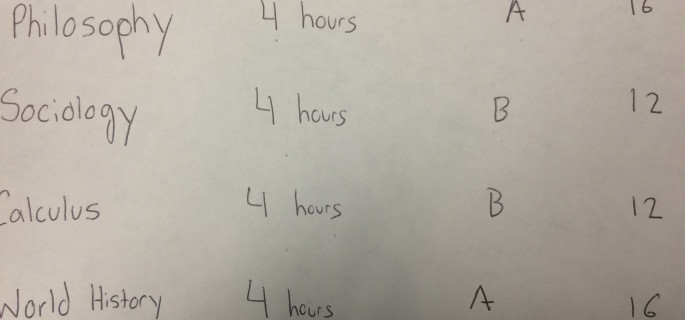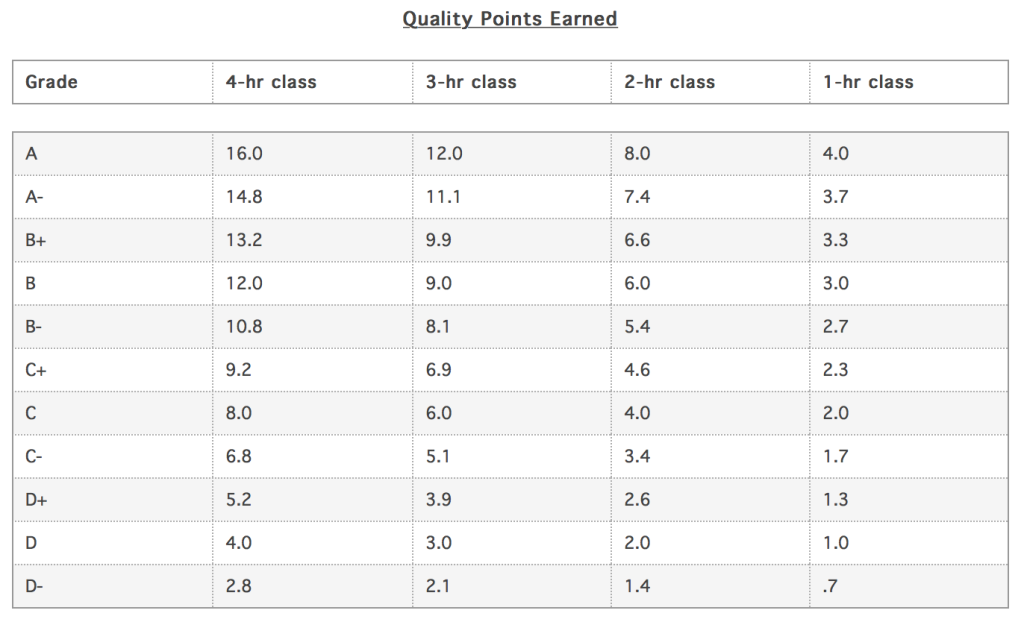Elon, North Carolina|Thursday, January 26, 2017

# Do it yourself: Calculating your cumulative GPA at ElonWhile midterms may have ended, the final exams are starting to become a reality for Elon students.

The thought of exams always brings anxiety for some students about the grades they need to keep up their grade point average goals. To give you a good idea of what your GPA would look like after your exams, here is an easy way to calculate your cumulative GPA.

First visit the Elon University Registrars Office website by clicking here. If you look to the left there will be a “GPA Calculation Formula” tab, click there to access the Quality Points Earned table. This table represents the number of quality points earned based on two factors: the grade you receive and the number of credit hours the class counts for.This Quality Points Earned table is a useful tool for students to quickly access point values for class grades

The students that receive an “A” in a four-credit-hour class, earn 16 quality points and the number of quality points earned for the same mark decrease by four points each time a credit-hour is taken away.

Now that you have calculated the total amount of quality points earned this semester, divide that by the number of credit-hours attempted for the semester.

Lets say that a student took four classes, each worth four credit hours, and received two “A”‘s and two “B”‘s. The quality points earned would add up to 56 and the number of credit-hours attempted would be sixteen.

The last step in this calculation is to divide the number of quality points by the number of hours attempted. In this scenario, the formula would be 56 divided by 16, which equates to a semester GPA of 3.5.

For the majority of students, this is not their first semester at Elon. To compute your cumulative GPA, add the new credit hours from this semester to any previous credit-hours at Elon and do the same for new quality points earned. Finally, divide the total number of quality points earned by the total number of hours attempted to receive the cumulative GPA.

The Elon 101 class taken by all first years is a special case when it comes to GPA calculation. If you pass your Elon 101 class it does not count towards your GPA. If you fail the Elon 101 class however, it will count towards your GPA as an “F”, so be careful!